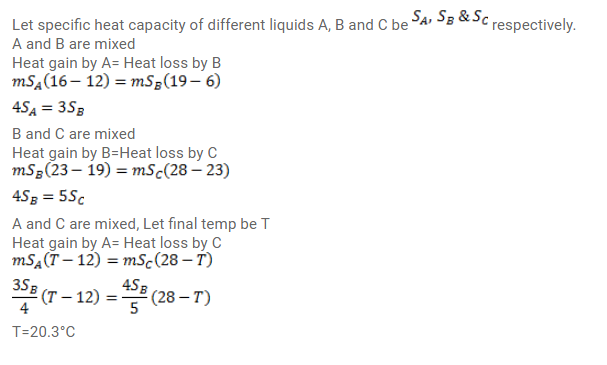# The temperatures of equal masses of three different liquidsQuestion:

The temperatures of equal masses of three different liquids are $\mathrm{A}, \mathrm{B}$ and $\mathrm{C}$ are $12^{\circ} \mathrm{C}, 19^{\circ} \mathrm{C}$ and $28^{\circ} \mathrm{C}$ respectively. The temperature when $\mathrm{A}$ and $\mathrm{B}$ are mixed is $16^{\circ} \mathrm{C}$, and when $\mathrm{B}$ and $\mathrm{C}$ are mixed, it is $23^{\circ} \mathrm{C}$. What will be the temperature when $A$ and $C$ are mixed?

Solution: